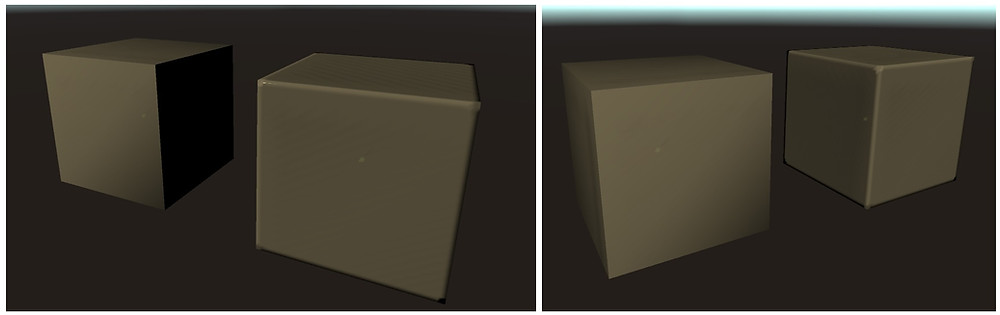# Mobile - friendly Bevel Shader (Unity)RecipeSimulating Bevel effect in Shader requires additional normal vectors for each vertex:

1. Smooth normal - calculated as if all vertices were shared between triangles.

2. Sharp normal - calculated normally, since all triangles are made of unique vertices.

3. 2 Normal vectors, one for each of triangle lines attached to the vertex.

4. Also one float3 is needed to package edge detection data.

Vertex count and triangle count remains the same. Transforming the mesh is a matter of selecting a correct mesh profile. In Profile image to the right you can see 3 LineNormals, it's because one of them will be replaced with Sharp Normal, but which one depends on in which position the given vertex is in a triangle. And the regular NORMAL is the smooth normal. My mesh editor has option to smooth the mesh without joining vertices - it calculates them as if they were one.

As mentioned before, "Smooth All" should be clicked to mark all vertices as smoothed for the Shader to work. And "All Unique" because every triangle has to consist of unique vertices.

Shaders and Mesh Packaging Profiles will be included with Playtime Painter. And also on my public GitHub, so no need to buy the Asset just for the Shaders. Also not sure if I can polish the usability of the mesh editor well enough by the next release.

Edge Strength, Atlas Texture and Color doesn't relate to this particular effect, but I believe it will make a nice synergy - I use colored edge to hide the seams. (Added bumped Bevel Shader below)

Update (2/12/2018)

The main downside was those nasty edges which end up looking in opposite direction from the camera. Quite often they are lit in a different way and become very visible. But problem became the solution: comparing them to camera view direction and clipping is easy:Video

ExamplesCode

The code for normal calculation is pretty lite:

```inline float3 DetectSmoothEdge(float3 edge, float3 junkNorm, float3 sharpNorm, float3 edge0, float3 edge1, float3 edge2) {
edge = max(0, edge - 0.965) * 28;

float allof = edge.r + edge.g + edge.b;
float border = min(1, allof);
float3 edgeN = edge0*edge.r + edge1*edge.g + edge2*edge.b;
float junk = min(1, (edge.g*edge.b + edge.r*edge.b + edge.r*edge.g)*2);
return   normalize((sharpNorm*(1 - border)+ border*edgeN)*(1 - junk) + junk*(junkNorm));
}```Below is a version of this Bevel shader that uses a Bump Map. I use edge detection to mask some seams with vertex color. Vertex color Alpha and Texture alpha are used as smoothness - that is why second image has more realistic reflections, but textures and meshes in both examples are the same.